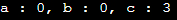# InClass Initializer and Delegation of Constructors in C++

## InClass Initializer and Delegation of Constructors in C++:

In this article, I am going to discuss InClass Initializer and Delegation of Constructors in C++ with Examples. Please read our previous article where we discussed Smart Pointers in C++ with Examples. These concepts are Introduced in C++ 11.

##### InClass Initializer and Delegation of Constructors in C++

Let us understand InClass Initializer and Delegation of Constructors in C++ with one example. Please have a look at the following code.

```class Test
{
int x = 15;
int y = 30;
};
```

Here, we have declared a class called Test and we have some variables and we directly assigned some values to them. This was not allowed in the previous version of C++. In C++ 11, we can directly assign the values to the member of our class. This is allowed in java and C#. So, the same thing is also allowed in C++ 11.

##### Example to Understand InClass Initializer in C++
```#include <iostream>
using namespace std;
class Test
{
int x = 15;
int y = 30;
public:
void Display ()
{
cout << "x : " << x << ", y : " << y;
}
};

int main()
{
Test obj;
obj.Display ();
}
```

Output: x : 15, y : 30

And if we have a constructor in class Test as follows,

```Test(int a, int b){
x = a;
y = b;
}
```

Here, we created one constructor which is taking two integer parameters and setting the values of x and y variables. Suppose, we have one more constructor which is a non-parameterized constructor which doesn’t take anything. Instead of writing the code for the constructor, we can simply delegate the constructor and pass parameter values as (1, 1).This non-parameterized constructor will call the above-parameterized constructor by passing the values (1, 1). For a better understanding, please have a look at the following example.

```#include <iostream>
using namespace std;
class Test
{
int x = 15;
int y = 30;
int z;
public:
Test(int a, int b)
{
x = a;
y = b;
}
Test():Test(35, 75)
{
z = 10;
}
void Display()
{
cout << "x : " << x << ", y : " << y << ", z : " << z;
}
};

int main()
{
Test obj;
obj.Display();
}```

Output: x : 35, y : 75, z : 10

Suppose we have written some logic for validation of data members inside the parameterized constructor, then we don’t have to repeat the logic. Instead of writing the logic again in the non-parameterized constructor, we can call the other constructor by passing the parameter. So, it means one constructor can call the other constructor within the same class. So, this is the delegation of the constructor. This is the new feature of C++11.

##### Example to Understand Delegation of Constructors in C++:
```#include <iostream>
using namespace std;
class MyClass
{
int a, b, c;

public:
MyClass()
{
a = b = c = 0;
}

//using constructor delegation
MyClass (int c): MyClass()
{
this->c = c;
}

void display()
{
cout << "a : " << a << ", b : " << b << ", c : " << c;
}
};

int main()
{
MyClass my_obj(3);
my_obj.display();
}
```
###### Output:In the next article, I am going to discuss Ellipsis in C++ with Examples. Here, in this article, I try to explain InClass Initializer and Delegation of Constructors in C++ with Examples and I hope you enjoy this article.